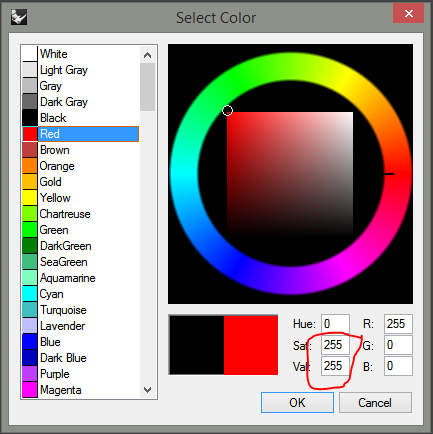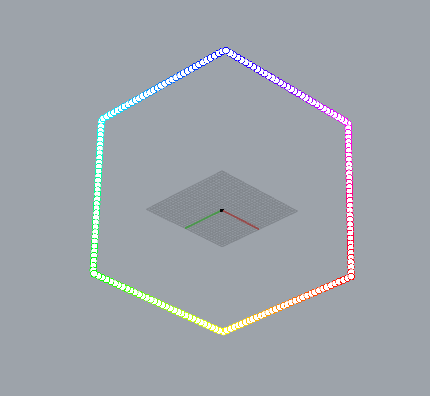# HSL to RGB conversion via RhinoCommon or (rhinoscriptsyntax)

I can’t seem to get this to work. rhinoscriptsyntax wants different stuff from RhinoCommon, but I can’t get either of them working…

``````import rhinoscriptsyntax as rs
import Rhino

count=5
hsl_colors=[Rhino.Display.ColorHSL(i/(count-1),1,1) for i in range(count)]
for color in hsl_colors: print "H={}, S={}, L={}".format(color.H,color.S,color.L)

rgb_colors=[Rhino.Display.ColorHSL.ToArgbColor(color) for color in hsl_colors]
for color in rgb_colors: print "R={}, G={}, B={}".format(color.R,color.G,color.B)
``````

Result:

``````H=0.0, S=1.0, L=1.0
H=0.25, S=1.0, L=1.0
H=0.5, S=1.0, L=1.0
H=0.75, S=1.0, L=1.0
H=1.0, S=1.0, L=1.0
R=255, G=255, B=255
R=255, G=255, B=255
R=255, G=255, B=255
R=255, G=255, B=255
R=255, G=255, B=255
``````

What am I doing wrong here?

Thanks,
–Mitch

Hi Mitch,

You only change the Hue which does not do much with a color that’s both fully saturated and light as that is white.

If you vary the other values in HSL color you get this result:

``````H=0.0, S=0.0, L=0.0
H=0.25, S=0.25, L=0.25
H=0.5, S=0.5, L=0.5
H=0.75, S=0.75, L=0.75
H=1.0, S=1.0, L=1.0
R=0, G=0, B=0
R=63, G=79, B=47
R=63, G=191, B=191
R=191, G=143, B=239
R=255, G=255, B=255
``````

-Willem

Hi Mitch,

The lightness is being set to the maximal value (1):

``````hsl_colors=[Rhino.Display.ColorHSL(i/(count-1),1,1) for i in range(count)]
``````

This is why all your RGB colors are white.
If you change it to some lesser value, your rgb colors will start getting their colors:

``````hsl_colors=[Rhino.Display.ColorHSL(i/(count-1),1,0.7) for i in range(count)]
``````

edit: Sorry for intruding Willem. Didn’t see your reply until I saved it (I am using 1024x768 screen resolution).

I always assumed that if the luminance was max and the saturation was max that you got the range of pure colors… Simply that the range was 0 to 1 and not 0-255…Guess that’s wrong then. How would you cycle through the 360° of the color wheel at full saturation then?

–Mitch

It’s not obvious but the type of color in that dialog is HS V not HS L

Set the Saturation to 1 and the Lightness to 0.5 to get the color wheel colors.

``````import rhinoscriptsyntax as rs
import Rhino

count=200
hsl_colors=[Rhino.Display.ColorHSL(i/(count-1),1,0.5) for i in range(count)]
for color in hsl_colors: print "H={}, S={}, L={}".format(color.H,color.S,color.L)

rgb_colors=[Rhino.Display.ColorHSL.ToArgbColor(color) for color in hsl_colors]
for color in rgb_colors:
rs.ObjectColor(point_obj,color)

``````-Willem

1 Like

OK, thanks much !! --Mitch

1 Like

If anyone wonders why I’m playing with this, I was just working on creating a function that will interpolate around the color wheel and return a list of n fully saturated colors. The easiest way to do this seemed to be to work with HSL colors - as they have the notion of saturation - and then convert back to RGB.

The function (below) could be used to color a series of objects or of layers, for example. You can interpolate a portion of the whole wheel by inputting a start value and and end value. Inputting a start/end range of 0 to 1 will interpolate HSL hues directly, otherwise inputting a range from 0 to 360 will convert Rhino color wheel “degrees” to RGB values - 0°/360° is Red, 120° is Green, 240° is Blue…

Anyway, FWIW here it is… --Mitch

``````import rhinoscriptsyntax as rs
import Rhino

def InterpolatedSatColorList(count,start,end):
"""returns an interpolated list of fully saturated RGB colors (via HSL). If
start and end are both between 0 and 1, input will be interpreted as the HSL
normalized domain; else as a degree value between 0 and 360 (color wheel)"""
if start<0 or start>360 or end<0 or end>360: return
if not(start<=1 and end<=1):
#remap from 0-360 to 0-1
start=start/360 ; end=end/360
inc=(end-start)/(count-1)
hsl_colors=[Rhino.Display.ColorHSL(start+(i*inc),1,0.5) for i in range(count)]
rgb_colors=[Rhino.Display.ColorHSL.ToArgbColor(color) for color in hsl_colors]
return rgb_colors

def TestFunction():
objs=rs.GetObjects("Select objects to color",preselect=True)
if not objs: return

start_hue=rs.GetReal("Enter start hue (0-360 or 0-1)",0,0,360)
if start_hue is None: return

end_hue=rs.GetReal("Enter end hue (0-360 or 0-1)",360,0,360)
if end_hue is None: return

color_list=InterpolatedSatColorList(len(objs),start_hue,end_hue)
rs.EnableRedraw(False)
for i,obj in enumerate(objs): rs.ObjectColor(obj,color_list[i])
TestFunction()
``````